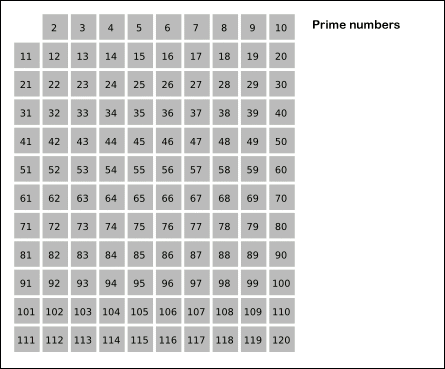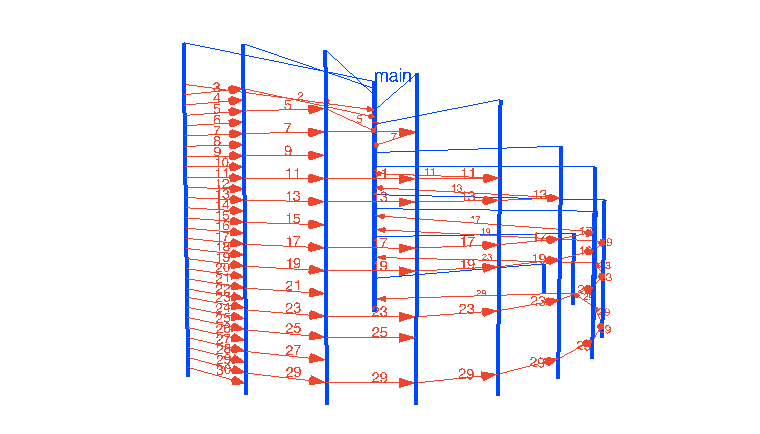# JAVA - 带你走近Go语言编程思维## 2.现实中的“投影”

• 问题描述：素数是一个自然数，它具有两个截然不同的自然数除数：1和它本身。这里的问题是如何找到小于或等于给定整数n的素数。针对这个问题，我们可以采用埃拉托斯特尼素数筛算法。

• 算法描述：先用最小的素数2去筛，把2的倍数剔除掉；下一个未筛除的数就是素数（这里是3）。再用这个素数3去筛，筛除掉3的倍数… 这样不断重复下去，直到筛完为止（算法图示见图1）。## 1）C语言版本

``````// sieve.c
#include <stdio.h>

#define LIMIT  50
#define PRIMES 10

void sieve() {
int c, i,j,numbers[LIMIT], primes[PRIMES];

for (i=0;i<LIMIT;i++){
numbers[i]=i+2; /*fill the array with natural numbers*/
}

for (i=0;i<LIMIT;i++){
if (numbers[i]!=-1){
for (j=2*numbers[i]-2;j<LIMIT;j+=numbers[i])
numbers[j]=-1; /* 筛除非素数 */
}
}

c = j = 0;
for (i=0;i<LIMIT&&j<PRIMES;i++) {
if (numbers[i]!=-1) {
primes[j++] = numbers[i]; /*transfer the primes to their own array*/
c++;
}
}

for (i=0;i<c;i++) printf("%d\n",primes[i]);
}
``````

``````// sieve.hs

sieve [] = []
sieve (x:xs) = x : sieve (filter (\a -> not \$ a `mod` x == 0) xs)
n = 100
main = print \$ sieve [2..n]
``````

## 3）Go语言版本

``````// sieve.go

func Generate(ch chan<- int) {
for i := 2; ; i++ {
ch <- i
}
}

func Filter(in <-chan int, out chan<- int, prime int) {
for {
i := <-in
if i%prime != 0 {
out <- i
}
}
}

func main() {
ch := make(chan int)
go Generate(ch)
for i := 0; i < 10; i++ {
prime := <-ch
print(prime, "\n")
ch1 := make(chan int)
go Filter(ch, ch1, prime)
ch = ch1
}
}
``````

• C版本的素数筛程序是一个常规实现。它定义了两个数组：numbers和primes，“筛”的过程在numbers这个数组中进行（即基于纯内存修改），非素数的数组元素被设置为-1，便于后续提取；

• Haskell版本采用了函数递归的思路，通过“filter操作集合”，用谓词（过滤条件）\a -> not \$ a `mod` x == 0；筛除素数的倍数，将未筛除的数的集合作为参数传递归递给下去；

• Go版本程序实现了一个并发素数筛，它采用的是goroutine的并发组合。程序从素数2开始，依次为每个素数建立一个goroutine，用于作为筛除该素数的倍数。ch指向当前最新输出素数所位于的筛子goroutine的源channel，这段代码来自于Rob Pike的一次关于并发的分享。Go版本程序的执行过程可以用图2立体的展现出来。## 3.Go语言原生编程思维

Go语言诞生较晚，大多数Gopher（包括笔者在内）第一语言都不是Go，都是“半路出家”从其他语言转过来的，如C、C++、Java、Python等。每种语言都有自己的原生编程思维。比如：C语言相信程序员，提供了指针和指针运算，让C程序员天马行空的发挥，接近底层的直接内存操作让C程序拥有很高的性能；C++支持多范式（命令式、OO和泛型），虽不强迫程序员使用某个特定的范式，但推荐使用最新代表现代语言发展特色的泛型等高级范式；Python语言更是形成了Pythonic规则来指导Python程序员写出符合Python思维或惯用法的代码。